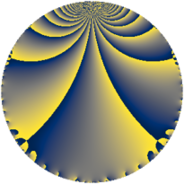# Properties

 Label 6384.2.a.kLevel $6384$ Weight $2$ Character orbit 6384.a Self dual yes Analytic conductor $50.976$ Analytic rank $1$ Dimension $1$ CM no Inner twists $1$

# Related objects

Show commands: Magma / PariGP / SageMath

## Newspace parameters

comment: Compute space of new eigenforms

[N,k,chi] = [6384,2,Mod(1,6384)]

mf = mfinit([N,k,chi],0)

lf = mfeigenbasis(mf)

from sage.modular.dirichlet import DirichletCharacter

H = DirichletGroup(6384, base_ring=CyclotomicField(2))

chi = DirichletCharacter(H, H._module([0, 0, 0, 0, 0]))

N = Newforms(chi, 2, names="a")

//Please install CHIMP (https://github.com/edgarcosta/CHIMP) if you want to run this code

chi := DirichletCharacter("6384.1");

S:= CuspForms(chi, 2);

N := Newforms(S);

 Level: $$N$$ $$=$$ $$6384 = 2^{4} \cdot 3 \cdot 7 \cdot 19$$ Weight: $$k$$ $$=$$ $$2$$ Character orbit: $$[\chi]$$ $$=$$ 6384.a (trivial)

## Newform invariants

comment: select newform

sage: f = N # Warning: the index may be different

gp: f = lf \\ Warning: the index may be different

 Self dual: yes Analytic conductor: $$50.9764966504$$ Analytic rank: $$1$$ Dimension: $$1$$ Coefficient field: $$\mathbb{Q}$$ Coefficient ring: $$\mathbb{Z}$$ Coefficient ring index: $$1$$ Twist minimal: no (minimal twist has level 3192) Fricke sign: $$1$$ Sato-Tate group: $\mathrm{SU}(2)$

## $q$-expansion

comment: q-expansion

sage: f.q_expansion() # note that sage often uses an isomorphic number field

gp: mfcoefs(f, 20)

 $$f(q)$$ $$=$$ $$q - q^{3} + q^{7} + q^{9}+O(q^{10})$$ q - q^3 + q^7 + q^9 $$q - q^{3} + q^{7} + q^{9} - 6 q^{11} + 4 q^{13} + q^{19} - q^{21} + 2 q^{23} - 5 q^{25} - q^{27} + 2 q^{29} - 4 q^{31} + 6 q^{33} + 10 q^{37} - 4 q^{39} - 6 q^{41} + q^{49} - 6 q^{53} - q^{57} - 4 q^{59} + 6 q^{61} + q^{63} - 2 q^{67} - 2 q^{69} + 8 q^{71} - 6 q^{73} + 5 q^{75} - 6 q^{77} + 6 q^{79} + q^{81} - 16 q^{83} - 2 q^{87} + 10 q^{89} + 4 q^{91} + 4 q^{93} - 8 q^{97} - 6 q^{99}+O(q^{100})$$ q - q^3 + q^7 + q^9 - 6 * q^11 + 4 * q^13 + q^19 - q^21 + 2 * q^23 - 5 * q^25 - q^27 + 2 * q^29 - 4 * q^31 + 6 * q^33 + 10 * q^37 - 4 * q^39 - 6 * q^41 + q^49 - 6 * q^53 - q^57 - 4 * q^59 + 6 * q^61 + q^63 - 2 * q^67 - 2 * q^69 + 8 * q^71 - 6 * q^73 + 5 * q^75 - 6 * q^77 + 6 * q^79 + q^81 - 16 * q^83 - 2 * q^87 + 10 * q^89 + 4 * q^91 + 4 * q^93 - 8 * q^97 - 6 * q^99

## Embeddings

For each embedding $$\iota_m$$ of the coefficient field, the values $$\iota_m(a_n)$$ are shown below.

For more information on an embedded modular form you can click on its label.

comment: embeddings in the coefficient field

gp: mfembed(f)

Label $$\iota_m(\nu)$$ $$a_{2}$$ $$a_{3}$$ $$a_{4}$$ $$a_{5}$$ $$a_{6}$$ $$a_{7}$$ $$a_{8}$$ $$a_{9}$$ $$a_{10}$$
1.1
 0
0 −1.00000 0 0 0 1.00000 0 1.00000 0
 $$n$$: e.g. 2-40 or 990-1000 Significant digits: Format: Complex embeddings Normalized embeddings Satake parameters Satake angles

## Atkin-Lehner signs

$$p$$ Sign
$$2$$ $$1$$
$$3$$ $$1$$
$$7$$ $$-1$$
$$19$$ $$-1$$

## Inner twists

This newform does not admit any (nontrivial) inner twists.

## Twists

By twisting character orbit
Char Parity Ord Mult Type Twist Min Dim
1.a even 1 1 trivial 6384.2.a.k 1
4.b odd 2 1 3192.2.a.n 1
12.b even 2 1 9576.2.a.i 1

By twisted newform orbit
Twist Min Dim Char Parity Ord Mult Type
3192.2.a.n 1 4.b odd 2 1
6384.2.a.k 1 1.a even 1 1 trivial
9576.2.a.i 1 12.b even 2 1

## Hecke kernels

This newform subspace can be constructed as the intersection of the kernels of the following linear operators acting on $$S_{2}^{\mathrm{new}}(\Gamma_0(6384))$$:

 $$T_{5}$$ T5 $$T_{11} + 6$$ T11 + 6 $$T_{13} - 4$$ T13 - 4 $$T_{17}$$ T17 $$T_{23} - 2$$ T23 - 2

## Hecke characteristic polynomials

$p$ $F_p(T)$
$2$ $$T$$
$3$ $$T + 1$$
$5$ $$T$$
$7$ $$T - 1$$
$11$ $$T + 6$$
$13$ $$T - 4$$
$17$ $$T$$
$19$ $$T - 1$$
$23$ $$T - 2$$
$29$ $$T - 2$$
$31$ $$T + 4$$
$37$ $$T - 10$$
$41$ $$T + 6$$
$43$ $$T$$
$47$ $$T$$
$53$ $$T + 6$$
$59$ $$T + 4$$
$61$ $$T - 6$$
$67$ $$T + 2$$
$71$ $$T - 8$$
$73$ $$T + 6$$
$79$ $$T - 6$$
$83$ $$T + 16$$
$89$ $$T - 10$$
$97$ $$T + 8$$